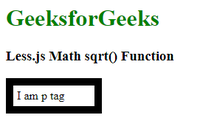Open in App
Not now

# Less.js Math sqrt() Function

• Last Updated : 11 Oct, 2022

Less.js is a simple CSS pre-processor that facilitates the creation of manageable, customizable, and reusable style sheets for websites. It is more advantageous because CSS employs a dynamic style sheet language. Several different browsers can use LESS because of its flexibility. In order for web browsers to use CSS, it must be built and enhanced using a computer language known as the CSS pre-processor. It is also a CSS language extension that provides tools like variables, functions, mixins, and operations to help create dynamic CSS while keeping backward compatibility.

In this article, we will discuss In this article, Math sqrt() function. The sqrt() function is used to find the square root of the passed number as a parameter.

Syntax:

`sqrt(number)`

Parameters:

• number: Any number (with or without a unit). If the unit is there then the output contains the same unit. For example: 25.0cm, 4px, 100 etc

Return type: The sqrt() functions returns a number.

Example 1: In this example, we have set the font size of the p tag using the sqrt() function.

The HTML code is as follows: (index.html)

## HTML

 ` ` `<``html` `lang``=``"en"``> ` ` `  `<``head``> ` `    ``<``link` `rel``=``"stylesheet"` `href``=``"/styles.css"``> ` ` ` ` `  `  ``<``body``> ` ` `  `    ``<``h1` `style``=``"color: green;"``>GeeksforGeeks ` `    ``<``h3``>Less.js Math sqrt() Function ` ` `  `    ``<``p``>My font size is: square root of 100px ` ` ` ` `  ``

Style.less

## CSS

 `p { ` `      ``font-size``: sqrt(``100px``); ` `}`

To compile the above LESS code into normal CSS, run the following command:

`lessc styles.less styles.css`

The compiled output CSS file looks like this:

styles.css

## CSS

 `p { ` `      ``font-size``: ``10px``; ` `}`

Output:Output of Less.js Math sqrt() Function example 1

Example 2: In this example, we have defined a variable @myvar, which stores the value of 25px. Now using the sqrt() method we have calculated the padding of the p tag by providing @myvar to it as an argument. Also, we have found out the width and border width of the p tag using the sqrt() function.

index.html

## HTML

 ` ` `<``html` `lang``=``"en"``> ` ` `  `<``head``> ` `    ``<``meta` `charset``=``"UTF-8"``> ` `    ``<``meta` `http-equiv``=``"X-UA-Compatible"`  `          ``content``=``"IE=edge"``> ` `    ``<``meta` `name``=``"viewport"`  `          ``content``=``"width=device-width, initial-scale=1.0"``> ` `    ``<``title``> ` `          ``Less.js Math sqrt() Function ` `    `` ` `    ``<``link` `rel``=``"stylesheet"` `href``=``"/styles.css"``> ` ` ` ` `  `<``body``> ` ` `  `    ``<``h1` `style``=``"color: green;"``>GeeksforGeeks ` `    ``<``h3``>Less.js Math sqrt() Function ` ` `  `    ``<``p``>I am p tag ` ` ` ` `  ``

styles.less

## CSS

 `@myvar: ``25px``; ` `p { ` `    ``width``: sqrt(``10000px``); ` `    ``border``: sqrt(``0.4``rem) ``solid` `black``; ` `    ``padding``: sqrt(@myvar); ` `}`

To compile the above LESS code into normal CSS, run the following command:

`lessc styles.less styles.css`

The compiled output CSS file looks like this:

styles.css

## CSS

 `p { ` `    ``width``: ``100px``; ` `    ``border``: ``0.63245553``rem ``solid` `black``; ` `    ``padding``: ``5px``; ` `}`

Output:Output of Less.js Math sqrt() Function

My Personal Notes arrow_drop_up
Related Articles